# Area Under a Curve - Day 1 of 2

4 teachers like this lesson
Print Lesson

## Objective

SWBAT use a sequence to estimate the area under a curve.

#### Big Idea

How can we find the area of highly irregular regions?

## Launch and Explore

15 minutes

The premise of today’s lesson is simple – find the area of a region bounded by a parabola. What students don’t know is that they will be using limits and that this is an introduction to very important work that they will do in calculus.

I start by giving students this worksheet and explaining that I would like them to find the area of the shaded region. If they cannot find the exact answer, I want them to get an estimate as accurate as they can. Then I let them loose and have them work with their table groups.

Here are some of the strategies that I usually observe when my students work on this:

1. Making a triangle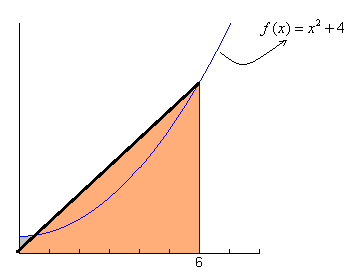2. Making a rectangle and a triangle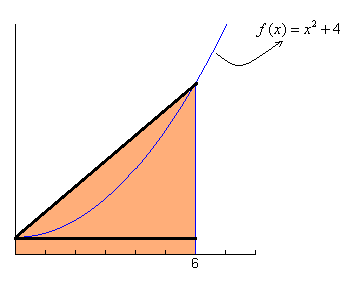3. Making rectangles inside the shaded region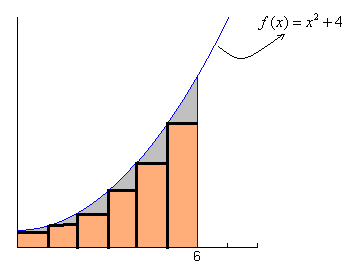4. Making rectangles outside the shaded region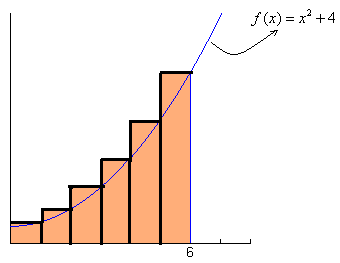5. Making trapezoids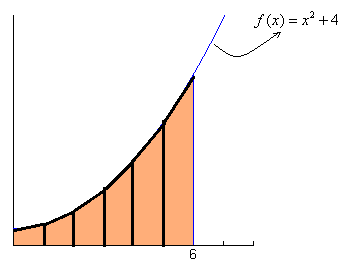If students don't do anything, I will ask them why it is so difficult to find the area of this region and they will say because it is so irregular. Then I ask them what they would do if it were a shape they were familiar with and they say they would use an area formula. So then I give them a hint to divide the region into shapes they do know in order to get an estimate.

If a group does get an estimate, then I will ask them to think about how they could get a more accurate estimate than what they already have.

## Share

20 minutes

After students have time to explore this problem, I will gather a few samples of student work and show them to the rest of the class to see if others can interpret their work. I will go from the least accurate estimate to the most accurate, so usually I start with the triangles then move on to the rectangles and then to the trapezoids. I don’t really worry about the calculations and numbers at this point, but rather focus on the geometry of dividing the region into other shapes.

On that first graph on the back of the worksheet we go through an finding a 6-rectangle estimate together. This will get all students on the same page of finding the area using rectangles. Teacher note: I choose the representation shown below with the rectangles outside of the region. Thus, when we write the area of n rectangle as a sequence, the last term will always have an x-value of 6, which will make our calculations simpler.After getting our initial estimate, I ask students how we can get a more accurate estimate of the area if we stick with the rectangles. Students usually suggest that we should find the estimate with the rectangles inside the unshaded region and then take the average of the two estimates. I tell them that this is a great idea but not the solution pathway I am looking for, so I will illicit more responses. It might take a minute for them to come up with making more rectangles, but they almost always come up with it. If my students do not bring it up on their own - I will usually ask some questions to guide them to that point.

Once we establish the need for more rectangles. I will have them write the area as the sum of 12 rectangles. Then I ask them to write it in summation notation and to calculate the estimate. I also ask them why making 12 rectangles is a better estimate of the actual area than 6 rectangles. Next, I say that we want to be even more accurate so I ask them to write the summation notation using 100 rectangles.

In this video I discuss how important Mathematical Practice #7 is in the this problem - making use of structure. Students may not be able to see the important patterns if we always write the final simplified base and height measurement for each rectangle.

## Extend

15 minutes

At this point I ask students how could we get a more accurate estimate than 100 rectangles and now they are on to me and know I want more rectangles. When I ask them how we could get the most accurate estimate, students are usually well aware that we need an infinite number of rectangles. Since we can’t literally calculate the area using an infinite number of rectangles, I ask what we should do instead. Usually at least one student will remember that we just used limits to find the value that a sequence is approaching, and that we are approaching the actual area of the region as the number of rectangles goes to infinity.

I challenge students to create an expression using a limit to find the exact area of the region. It is a little challenging since there are now two variables, so it might be helpful to start writing out the terms of the sequence for n rectangles to establish a pattern and see the structure (MP7) of the sequence.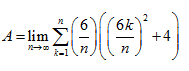The final equation for area is shown above. To close the lesson I tell students that this is one example where we can use limits that to find something that we would not have normally been able to calculate. Limits are powerful and we will continue to use them as we work on the road towards calculus.